Company Analysis

Access Bank Plc 2019FY: Robust Growth In Key Indices, But Assets Can Still Sweat Out

Current Market Price: N5.50
NPL RATIO (Q4-2019): 5.79%
LOAN to DEBT Ratio:56.31%
Year High: N12.00
Year Low: N5.30
Fair Value: N9.71
Equity Analyst: Tunde Segun Jeariogbe

Introduction
• In this report, we observed the audited financials of Access Bank Plc for the full-year ended December 31, 2019. The result was compared to numbers from the corresponding period of 2018 and thereafter established growth, projected expected earnings and performed valuation analysis accordingly.
• Although the bank effectively built its statement of comprehensive income items over the comparable year, its adoption of the new Finance Act 2019, which introduced a higher tax rate which depressed the Profit for the period, bringing it close to last year’s level.
• According to the financials, an assessment was carried out on the banks for the year under review to identify areas of uncertainty in tax treatment in accordance with IFRIC 23.
• Thus, some changes made to the Company Income Tax were brought about by the New Finance Act, which addresses the areas of losses of a capital nature, expenses incurred for the purpose of deriving tax-exempt income, taxes or penalties borne on behalf of another person and other changes as can be seen from the standard.
• Please understand that different shares outstanding were used to calculate the ratios in this report, due to the change experienced at the end of the merger with Diamond Bank Plc. In 2018, while shares outstanding stood at 28,927,971,631 units, it increased to 35,545,225,622 shares thereafter.
• Nevertheless, the board proposed a Cash Dividend of N0.40 per share, in addition to the previously distributed 25k Interim Cash Dividend.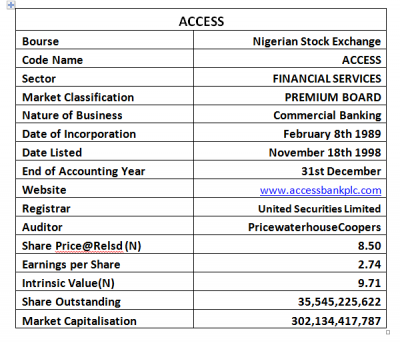Company figures
• Gross Earnings estimate for the year 2019 is valued at N666.75 billion, which is 26.10% above the N528.74 billion achieved at the end of 2018 full-year.
• Interest Income is valued at N536.84 billion, the same as 40.94% above the N380.91 billion in the corresponding year.
• Interest Expenses however increased by 25.22% to N259.61 billion up from the N207.33 billion stated in the 2018 financial report.
• Thus, Net Interest Income is estimated at N277.22 billion, versus N173.57 billion in 2018.
• With the bank further establishing its new growth status post-acquisition, Operating Expenses improved to N228.06 billion, same as N177.62 billion in the similar period of 2018.
• Thus, Profit before Tax grew from N103.18 billion of 2018 to N115.37 billion in the period.
• Having accounted for the Tax Expenses as noted above, the management of Access Bank achieved a marginal growth of 2.66% in the Profit for the year to N97.50 billion, versus N94.98 billion in 2018.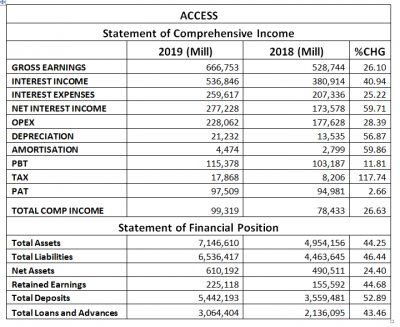• Access Bank’s Total Assets Valuation improved by 44.25% to N7.14 trillion, compared to N4.95 trillion stated in the 2018 financial report.
• Total Liabilities equally grew by 46.44% from the previously stated N4.46 trillion to N6.53 trillion.
• Net Assets also appreciated to N610.19 billion, compared to N490.51 billion in 2018.
• Retained Earnings improved in the same trend as it is currently valued at N225.11 billion, as against N155.59 billion in 2018.
• Further reflecting its new merger status, it achieved 52.89% growth in the Total Deposits through the year at N5.44 trillion, compared to N3.55 trillion in 2018.
• Thus, Total Loans and Advances through the just concluded financial year stood at N3.06 trillion, higher than the N2.13 trillion reported through 2018 financial year
Dividend Details
• As noted above, the company proposed a final dividend of N0.40 for the year ended 31st December 2019, which is 60.00% above the N0.25 paid in the corresponding year.
• The said N0.40 is in addition to the previously paid N0.25 interim dividend in the half-year 2019.
• Thus, total dividend paid through the year is the same as N0.65, as against N0.50 in the corresponding year.
• Annual Pay-Out Ratio is 23.69% higher than the previous year’s payout of 15.23%.
• Note that as against the market price of Access Bank when the result was released to the investing public, the said N0.40 cash dividend produced a 4.71% yield.
• Meanwhile, the Total Dividend paid for the year is 7.65% yield of the share price of Access Bank on the floor of the exchange when the result was released.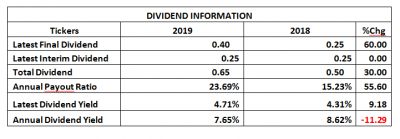Financial Strength/Solvency Ratios
• Just like other financial institutions, Access Bank’s Debt Ratio remained high at 0.91xmildly above the prior year’s estimate. In other words, Total Liabilities estimate for the period is the same as 91% of Total Assets valuation. Note that Total Deposit was added to the Liabilities figure used in the estimate.
• We also ascertained that the Total Debt for the period can replicate Equity 10.71 times, which is an improved position above the 9.10 times estimated in 2018. It is also important to understand that this ratio is typical of financial institutions.
• Total Equity was estimated at 0.09% of the Asset Value at the end of the year, which is below the 0.10% estimated in 2018.
• We have estimated a Beta value above the market’s Beta for Access Bank Plc shares. Also noteworthy is the fact that at 1.59 Beta value, it is above the industry average of 1.52.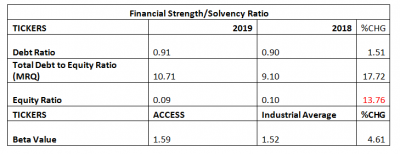Profitability Ratios
• Pre-Tax Margin stood at 17.30%, down from the previous year’s estimate of 19.52% for reasons earlier explained in the introductory paragraphs.
• Interest Expenses posted for the year is the same as 38.94% of the Estimated Gross Earnings, which is a marginal reduction from the 2018 estimate of 39.21%.
• Return on Average Equity used through the year was estimated at 15.98%, below the 19.36% achieved in 2018 numbers.
• See the table below for detailed profitability ratios and comparison.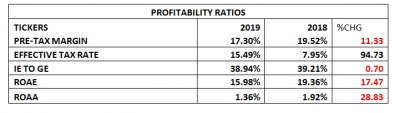Efficiency Ratios
• Operating Expense is estimated at 34.20% of the Gross Earnings Value estimate for the year, this is a marginal improvement in efficiency when compared to the 33.59% estimated from 2018 financial statistics.
• Similarly, Gross Earnings was estimated at 9.33% of Total Assets, a 12.18% drop in efficiency when compared to that of the corresponding year.
• Total Loan to Deposit Ratio achieved at the end of the year stood at 56.31%, down from the 60.01% achieved in 2018. Note that this is below the Central Bank of Nigeria’s mandated 65% for all financial institutions.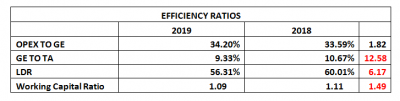Investment/Valuation Ratios
• At the end of the 2019 financial year, Access Bank earned N2.74 on each unit share, due to the difference in the shares outstanding between both years being compared in this report. The said earnings per share stood below the comparable year by 16.45%.
• Nevertheless, Total Comprehensive Income inched up by 3.06% to N2.79, as against the previous year’s N2.71 each.
• P/E-Ratio increased to 3.10x versus 1.77x in the corresponding year.
• The current Earnings per Share is the same as 32.27% of the share price of Access Bank at the time the result was released.
• The estimated value of each unit of Access Bank in its book is N17.17, a mild improvement above the N16.96 estimated in 2018.
• Confirming the under-priced status of Access Bank’s share price is the Price to Book Value estimate that stood below unity.
• See below for details.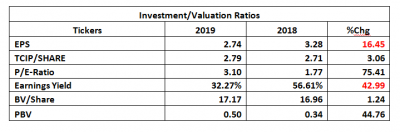Valuation
While valuing Access Bank’s shares, we choose a highly conservative method, seeking to present a very secure price to our users. Thus, we have valued each unit of Access Bank at N9.71, which is clearly below the Book Value but above the current market price of Access Bank on the Nigerian Stock Exchange. Hence, we have rated Access Bank a Buy.

Check Also
Close